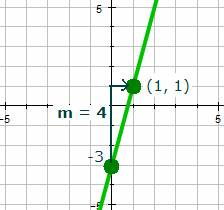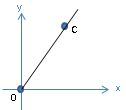# Slope Intercept Equation of a Line

Graphing a Line Using Its Slope and y-Intercept

The equation of a line with a slope of m and a y-intercept of (0, b) is y = mx + b.

To graph a line that is written in slope-intercept form:

1. Plot the y-intercept on the coordinate plane.
2. Use the slope to find another point on the line.
3. Join the plotted points with a straight line.

Example:

Graph the line. y = 4x – 3

Solution:

This line is given in slope-intercept form: y = mx + b.

The y-intercept of the line is –3. b = –3

The slope of the line is 4. m = 4

Therefore the graph of the equation is shown below.Example.

Determine the equation of the graph that is shown.Solution:

The y-intercept of the line is 1. b = 1.

The slope of the line is –2/3. m = –2/3.

Substitute these values into the slope-intercept equation of a line: y = mx + b

The equation of the line is $y = -\frac{2}{3}x + 1$.In the xy-plane above, point C has coordinates (6, 9). Which of the following is an equation of the line that contains points O and C?The graph shown above gives the relation between x° F and y° C. Which equation is represented by the graph?

The y-intercept of 4x - 2y = 8 is